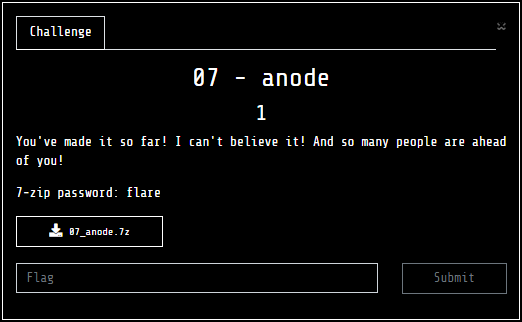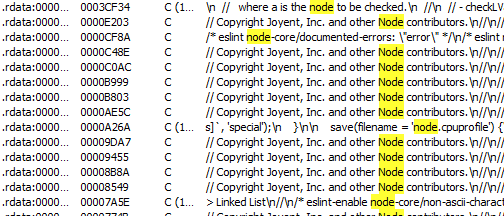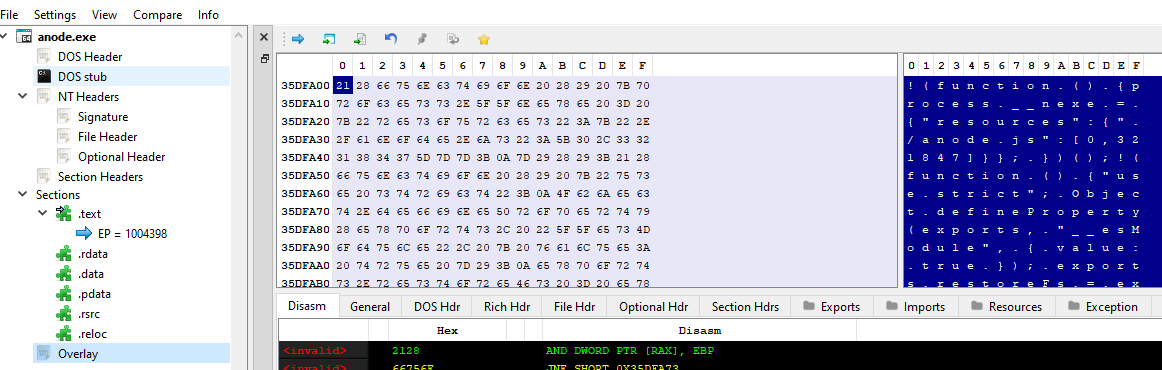# Writeup: FlareOn 2022: 007 - anode## 1. TLDR## 2. Input data

The challenge file is here. Password: flare.

The subject of the task was the PE file:

``````anode.exe
``````

## 3. Analysis of the file as a PE file

I verified the file type:

``````\$ file anode.exe
anode.exe: PE32+ executable (console) x86-64, for MS Windows
``````

The program displayed a message after starting:

``````Enter flag:
``````

After typing a string, the program terminated.

I loaded the program into IDA. I noticed many strings indicating that it was a program using node.js:Using the pe-bear tool, I identified an overlay containing code written in javascript:Reading the `anode.exe` file from byte `35dfa00`, I saved the javascript to a separate file and started analyzing it:

``````
readline.question(`Enter flag: `, flag => {
if (flag.length !== 44) {
console.log("Try again.");
process.exit(0);
}
var b = [];
for (var i = 0; i < flag.length; i++) {
b.push(flag.charCodeAt(i));
}

// something strange is happening...
if (1n) {
console.log("uh-oh, math is too correct...");
process.exit(0);
}

var state = 1337;
while (true) {
state ^= Math.floor(Math.random() * (2**30));
switch (state) {
case 306211:
if (Math.random() < 0.5) {
b -= b + b + b + b + b + b + Math.floor(Math.random() * 256);
b &= 0xFF;
} else {
b -= b + b + b + b + b + b + 225;
b &= 0xFF;
}
state = 868071080;
continue;
case 311489:
if (Math.random() < 0.5) {
b -= b + b + b + b + b + b + 115;
b &= 0xFF;
} else {
b ^= (b + b + b + b + b + b + 19) & 0xFF;
}
state = 22167546;
continue;

...

case 185078700:
break;

...

case 1071767211:
if (Math.random() < 0.5) {
b ^= (b + b + b + b + b + b + 241) & 0xFF;
} else {
b ^= (b + b + b + b + b + b + 37) & 0xFF;
}
state = 109621765;
continue;
default:
console.log("uh-oh, math.random() is too random...");
process.exit(0);
}
break;
}

var target = [106, 196, 106, 178, 174, 102, 31, 91, 66, 255, 86, 196, 74, 139, 219, 166, 106, 4, 211, 68, 227, 72, 156, 38, 239, 153, 223, 225, 73, 171, 51, 4, 234, 50, 207, 82, 18, 111, 180, 212, 81, 189, 73, 76];
if (b.every((x,i) => x === target[i])) {
console.log('Congrats!');
} else {
console.log('Try again.');
}
});
``````

## 4. Analysis of javascript code

The script took a flag as input and then checked if it was correct by calculating a “checksum.” Attempting to run the slightly revised code in Chrome resulted in an output:

``````uh-oh, math.random() is too random...y
``````

My attention was drawn to the comment `// something strange is happening...` and the if statement next to it, which should always terminate the program. So, either the script itself should have been analyzed (bypassing the “malicious” code fragments) or the javascript interpreter was acting differently than expected. In order to confirm this hypothesis, I tried to analyze the interpreter’s code snippet after giving text to the standard input. After a few minutes, I concluded that a thorough analysis and revealing the interpreter’s “cheating” would be too breakneck. So I decided to copy the `anode.exe` file and overwrite the javascript code snippet so as to distinguish between the different code fragments.

To do this, I overwrote the first of the strings `Try again` to `Wrong len`.

I then conducted 2 experiments:

I entered a string of incorrect length into the input:

``````>myanode.exe
Enter flag: aa
Wrong len.
``````

I then input a string of characters of the correct length (44 characters):

``````>myanode.exe
Enter flag: aaaaaaaaaaaaaaaaaaaaaaaaaaaaaaaaaaaaaaaaaaaa
Try again.
``````

It seemed that the if statements were working in reverse. To confirm, I made one more change:

``````// something strange is happening...
if (true){
console.log("uh-oh, math is too correct...")
``````

The standard output showed:

``````>python -c "print('a'*44)" | .\myanode.exe
Enter flag: aaaaaaaaaaaaaaaaaaaaaaaaaaaaaaaaaaaaaaaaaaaa
uh-oh, math is too correct...
``````

In the reverse case:

``````// something strange is happening...
if (false){
console.log("uh-oh, math is too correct...")
``````

… a message indicating further execution could be observed:

``````>python -c "print('a'*44)" | .\myanode.exe
Enter flag: aaaaaaaaaaaaaaaaaaaaaaaaaaaaaaaaaaaaaaaaaaaa
Try again.
``````

With the knowledge I gained, I decided to test the Math.floor() and Math.random() functions. As a result of the experiment, I determined that each time, the first execution of Math.random() gives the same result.

## 5. Deobfuscation

Knowing that the generator seed is fixed, I proceeded to deobfuscate. I decided to generate the states that the program goes through. To do this, I re-wrote the `// something strange is happening...` comment, the accompanying `if` statement with code and the beginning of the while loop in such a way as to trace the execution through the various case blocks:

``````var state = 1337;
while (true) {
state ^= Math.floor(Math.random() * (2**30));
console.log(state);
switch (state) {
``````

I then ran the code and generated a list of states:

``````>python -c "print('a'*31+'@flare-on.com')" | .\myanode.exe > states.txt
``````

In the next step, I developed a tool with which:

1. I identified the individual if/else blocks that were executed by the interpreter
2. I calculated the subsequent elements of the string generated by the PRNG
``````#!/usr/bin/python3
import re
import time
from subprocess import Popen, PIPE,STDOUT
from tqdm import tqdm

def get_content(filename):
content = None
with open(filename, 'rb') as f:
return content

def get_lines(filename):
return get_content(filename).decode().split('\n')

def get_start_code(script):
start_code = ''
start_line = 0
for i in range(len(script)):
if "var state" in script[i]:
start_code += script[i]
if "state ^= Math" in script[i]:
start_code += script[i]
start_line = i+1
start_code += r'''console.log(state);'''
return start_code, start_line

def save_code(code, filename):
with open(filename, 'wb') as script:
script.write(code.encode())

def patch_file(filename, offset, code):
with open(filename, 'rb+') as exe:
exe.seek(offset, 0)
exe.write(code.encode())

def copy_file(src,dst):
content = None
with open(src, 'rb') as s:
with open(dst, 'wb') as d:
d.write(content)

def execute_in(executable, patch_offset, patch_max_length, code, line=-1):
if len(code)>patch_max_length:
chars_to_remove = len(code) - patch_max_length
lines = code.split('\n')
nchars_to_remove = (0 if (len(lines)>chars_to_remove) else chars_to_remove-len(lines))
lines=' '*(len(lines)-chars_to_remove)
chars_to_remove = nchars_to_remove
if chars_to_remove>0:
for i in reversed(range(len(lines))):
if re.match(r'//', lines[i]):
if len(lines[i])<=chars_to_remove:
chars_to_remove-=len(lines[i])
lines[i] = ''
else:
lines[i]=' '*(len(lines[i])-chars_to_remove)
chars_to_remove=0
if chars_to_remove==0:
break
code='\n'.join(lines)

if len(code)>patch_max_length:
print(f"!!!ERROR!!! New code length is not equal to original code! Expected: {patch_max_length}. Given: {len(code)}")
exit(1)
code += (' '*(patch_max_length - len(code)))

tmp_exe = executable.replace('.exe', f'{line}.exe')
copy_file(executable, tmp_exe)
patch_file(tmp_exe, patch_offset, code)
p = Popen([tmp_exe], stdout=PIPE, stdin=PIPE, stderr=STDOUT)
default_input = b'b'*44+b'\n'
output = p.communicate(input=default_input)
p.kill()
result = output.decode().split('\n').replace("Enter flag: ","").strip()
time.sleep(0.05)
return result

def look_for(script, start_line, text):
result = None
for i in range(start_line, len(script)):
if text in script[i]:
result = i
break
return result

def get_script_lines(filename, offset, length):
content=None
with open(filename,'rb') as f:
f.seek(offset, 0)
content = content.decode().split('\n')
return content

def evaluate(code_lines, line_number, executable,script_offset,script_length, math_floor_expression):
expression = code_lines[line_number].replace(math_floor_expression, "kv")
code_lines[line_number] = f"var kv={math_floor_expression};{expression}console.log(kv);"
known_value = execute_in(executable,script_offset,script_length, '\n'.join(code_lines))
code_lines[line_number] = expression.replace("kv", known_value)
return code_lines[line_number]

def main():

### settings ###
script_offset = 0x35E3964
script_end = 0x363213C
script_length = script_end-script_offset
math_floor_expression = "Math.floor(Math.random() * 256)"
executable = '.\\anode_interpreter.exe'
################

script = get_script_lines(executable, script_offset, script_length)
states = get_lines(".\states.txt")

start_code, start_line = get_start_code(script)

output=''

for state_counter in tqdm(range(len(states))):
state = states[state_counter]
case_start = look_for(script,start_line,state)
if not case_start:
print("Output:")
print(output)
exit(1)
continue_start = "break" not in script[case_start+1]
if continue_start:
if_start = case_start+1
if_statement = script[if_start]
else_start = look_for(script, if_start, "} else {")
state_start = look_for(script, case_start, "state = ")
if_script_block = '\n'.join(script[if_start+1:else_start])
else_script_block = '\n'.join(script[else_start+1:state_start-1])
state_log_script = script.copy()
state_log_script[else_start-1] += r'''console.log('True');'''
state_log_script[state_start-2] += r'''console.log('False');'''
test_result = ("True" == execute_in(executable,script_offset,script_length, '\x0A'.join(state_log_script)))
state_log_script = script.copy()
if test_result:
if math_floor_expression in if_script_block:
for i in range(if_start+1, else_start):
if math_floor_expression in state_log_script[i]:
state_log_script[i] = evaluate(state_log_script,i, executable,script_offset,script_length, math_floor_expression)
break
output+='\n'.join(state_log_script[if_start+1:else_start])+'\n'
else:
if math_floor_expression in else_script_block:
for i in range(else_start+1,state_start-1):
if math_floor_expression in state_log_script[i]:
state_log_script[i] = evaluate(state_log_script,i, executable,script_offset,script_length, math_floor_expression)
break
output+='\n'.join(state_log_script[else_start+1:state_start-1])+'\n'

else:
look_for(script, case_start, "break;")
break

save_code(output, 'output.js')

if __name__=="__main__":
main()
``````

As a result of the script, I obtained a `output.js` file containing 1024 assignments. After delicate editing, involving the removal of “noise” generated by actions modulo 255 from the following lines, I obtained a set of consecutive assignments:

``````b -= b + b + b + b + b + b + 7;
b += b + b + b + b + b + b + 79;
b ^= b + b + b + b + b + b + 255;
b ^= b + b + b + b + b + b + 245;
b += b + b + b + b + b + b + 177;
b -= b + b + b + b + b + b + 151;
...
b += b + b + b + b + b + b + 49;
b ^= b + b + b + b + b + b + 213;
b -= b + b + b + b + b + b + 185;
b += b + b + b + b + b + b + 179;
b += b + b + b + b + b + b + 14;
b += b + b + b + b + b + b + 50;
b += b + b + b + b + b + b + 36;
``````

Therefore, it remained to reverse the order of the assignments performed and read the flag. To do this, I wrote a script:

``````#!/usr/bin/python3

def get_content(filename):
content = None
with open(filename, 'rb') as f:
return content

def get_lines(filename):
return get_content(filename).decode().split('\n')

def parse_index_from(variable):
try:
index = int(variable.strip()[2:][:-1])
except ValueError:
index = None
return index

def find_operator(line, operations):
result = None
for operator in operations.keys():
if f"{operator}" in line:
result = operator
break
return result

def process(line, b, operations):
operator = find_operator(line, operations)
equation = line.replace(";","").split(operator)
variable = equation.strip()
variable_index=parse_index_from(variable)
operands = equation.split("+")
total = 0
for operand in operands:
stripped_operand = operand.strip()
operand_index = parse_index_from(stripped_operand)
total += (b[operand_index] if operand_index !=None else int(stripped_operand))
b[variable_index] = (operations[operator](b[variable_index], total) & 0xFF)
return b

def main():
b = [106, 196, 106, 178, 174, 102, 31, 91, 66, 255, 86, 196, 74, 139, 219, 166, 106, 4, 211, 68, 227, 72, 156, 38, 239, 153, 223, 225, 73, 171, 51, 4, 234, 50, 207, 82, 18, 111, 180, 212, 81, 189, 73, 76]
lines = get_lines(".\equations.txt")
operations = {"+=": (lambda a,t : a-t),\
"-=": (lambda a,t : a+t),\
"^=": (lambda a,t : a^t)}

for i in reversed(range(len(lines))):
b = process(lines[i], b, operations)
flag = ''.join([chr(c) for c in b])
print(flag)

if __name__ == "__main__":
main()
``````

After running, a flag appeared on the standard output:

``````n0t_ju5t_A_j4vaSCriP7_ch4l1eng3@flare-on.com
``````